# Well Control Formulas Part 6

This part of well control formulas will demonstrate you more about some equations frequently used in snubbing operation. In Well Control Formulas Part 5, I show just only some snubbing formulas and this part will show the rest of them.

I wish you would enjoy reading my blog post.

Buoyed Weight of Open Ended Tubulars (Wb),lb

For this formula, you just use the buoyancy factor and multiply it with weight of pipe in the air.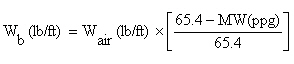Buoyed Weight of Closed Ended Tubulars without fluid in the pipe (Wb),lb

This formula below is different from the 1st formula because it is used for the closed ended pipe. Weight in the air will be subtracted with buoyancy weight of pipe ran in hole.

Note: 24.5 is conversion factor to make the unit in gallon.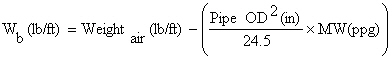Buoyed Weight of Closed Ended Tubulars after filling the pipe (Wb),lb -> in this case, there is different fluid weight in pipe and annular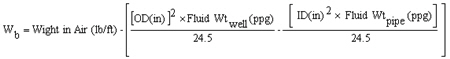The Balance Point for closed ended and unfilled pipe is the point where the weight of pipe in the fluid equates to force created by wellhead pressure.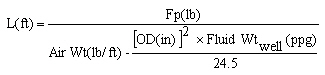Note:ORThis is the second case for balance point calculation. The Balance Point for closed ended  pipe and the pipe is filled with fluid.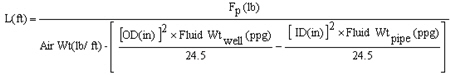Maximum Down Force on Jacks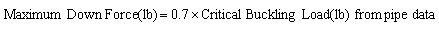Effective Area of Snubbing Jacks, square inch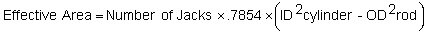Hydraulic Pressure to Snub, psi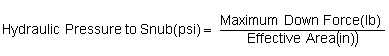Share the joy